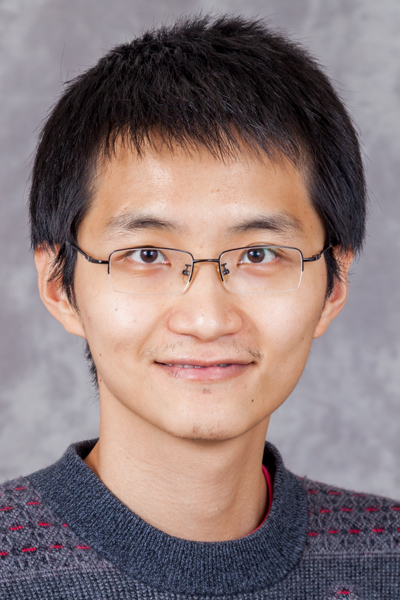Home

•论机器学习中鲁棒性和博弈论：非凸优化与矩阵完成

Speaker

Yu Cheng, Duke UniversityTime

2019-01-04 14:00:00 ~ 2019-01-04 15:30:00

Location

Room 3-412, SEIEE Building

Host

Weinan Zhang、Yuye Ling

Abstract
Matrix completion is a well-studied problem with many machine learning applications In practice, the problem is often solved by non-convex optimization algorithms However, the current theoretical analysis for non-convex algorithms relies heavily on the assumption that every entry is observed with exactly the same probability p, which is not realistic in practice In this paper, we investigate a more realistic semi-random model, where the probability of observing each entry is at least p Even with this mild semi-random perturbation, we can construct counter-examples where existing non-convex algorithms get stuck in bad local optima In light of the negative results, we propose a pre-processing step that tries to re-weight the semi-random input, so that it becomes "similar " to a random input We give a nearly-linear time algorithm for this problem, and show that after our pre-processing, all the local minima of the non-convex objective can be used to approximately recover the underlying ground-truth matrix This is a joint work with Rong Ge The paper is available at: https: arxiv org abs 1803 10846
Bio
Yu Cheng is a postdoc in the Computer Science Department at Duke University. He obtained his Ph.D. in Computer Science from University of Southern California in 2017. He is interested broadly interested in theoretical computer science, in particular problems at the intersection of learning theory and game theory. Homepage: https://users.cs.duke.edu/~yucheng/
© John Hopcroft Center for Computer Science, Shanghai Jiao Tong University